## Figures index

#### František Trebuňa, František Šimčák, Miroslav Pástor

American Journal of Mechanical Engineering. 2016, 4(7), 266-270 doi:10.12691/ajme-4-7-7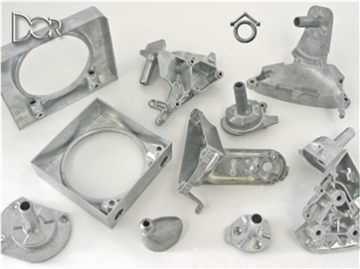• Figure 1. Moulds from aluminum alloys produced by high pressure casting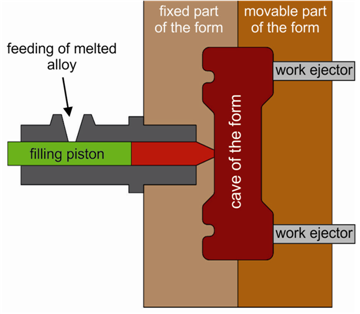• Figure 2. Principle of high-pressure die casting• Figure 3. Die casting machine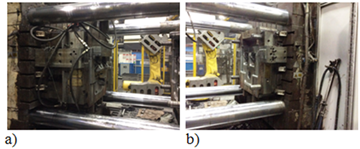• Figure 4. View of the movable (a) and stationary (b) sections of the die casting mold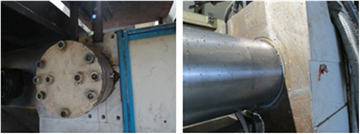• Figure 5. Fixing the nut of the guide column to the stationary section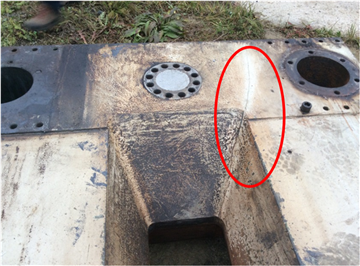• Figure 6. Stationary plate with a crack• Figure 7. Areas of hardness measurement from the side of the casting mold (I and II)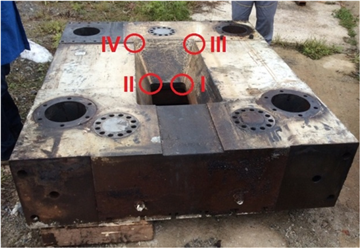• Figure 8. Areas of measurement from the exterior side (III and IV)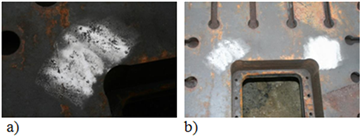• Figure 9. Preparation of areas for hardness measurement• Figure 10. Performing hardness measurement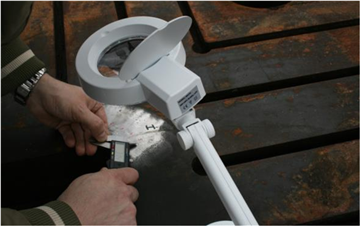• Figure 11. Reading of the indentation diameter on the tested plate (area I, point 5) using a digital gauge and a magnifier• Figure 12. Standard test bars a) marking 73, areas I, II, IV, b) marking 72, area III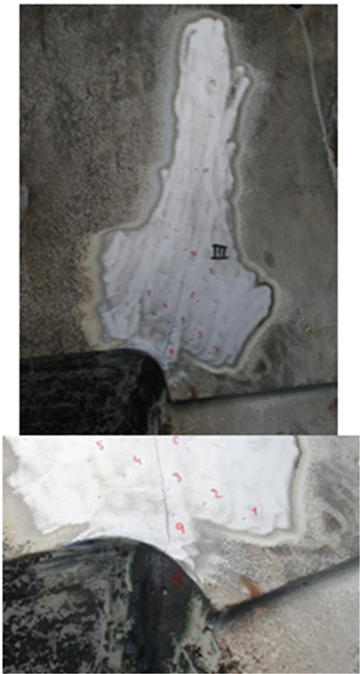• Figure 13. Points of hardness measurement in area III – crack neighborhood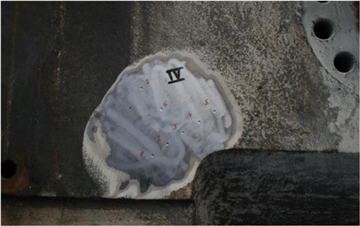• Figure 14. Points of hardness measurement in area IV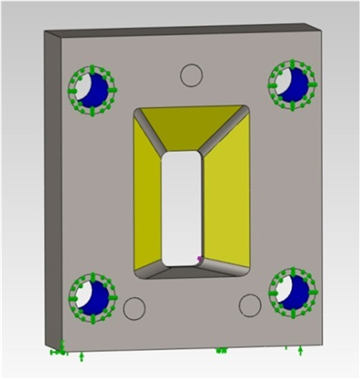• Figure 15. Model of the stationary plate with boundary conditions - uniform transfer of forces by individual columns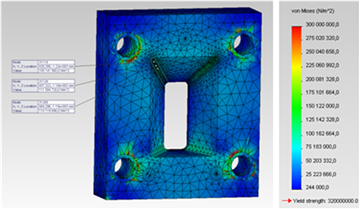• Figure 16. Field of equivalent stresses with the uniform transfer of forces by individual guide columns